Next: MDPs in Varying Environments Up: Generalized Markov Decision Processes Previous: Generalized Markov Decision Processes

### Q-learning in Generalized MDPs

As shown by Szepesvári and Littman, the analogue of the Q-learning algorithm (Section 2.1) can be defined in generalized MDPs as well [Szepesvári and Littman(1996)]. Furthermore, convergence results for this general algorithm can also be established.

In this subsection we review the generalized algorithm. We restrict ourselves to models where operator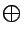is the expected value operator, i.e.,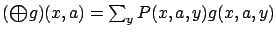. This simplifies the definition considerably, and for the purposes of this article this special case is sufficient. The general definition can be found in the work of [Szepesvári and Littman(1996)].

In Q-learning, for the optimal state-action value function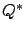,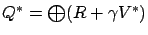holds. Note thatis the fixed point of operator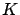, which is defined by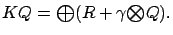(2)

The generalized Q-learning algorithm starts with an arbitrary initial value function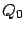, and then uses the following update rule: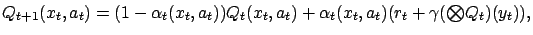(3)

where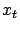is the current state,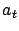is the selected action,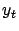is the resulting state,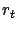is the gained reward, and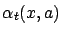is the learning rate at time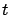.is selected according to the probability distribution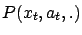and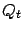is the actual estimate of.

It has been proven that under appropriate conditions on the order of updates and the learning parameters, the above Q-learning algorithm converges to the optimal Q-value function. The proof is based on a theorem that states the convergence of a specific asynchronous stochastic approximation. Both theorems are cited in Appendix A.Next: MDPs in Varying Environments Up: Generalized Markov Decision Processes Previous: Generalized Markov Decision Processes